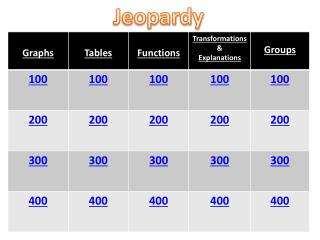DownloadDownload PresentationJeopardy

# Jeopardy

Download Presentation## Jeopardy

- - - - - - - - - - - - - - - - - - - - - - - - - - - E N D - - - - - - - - - - - - - - - - - - - - - - - - - - -
##### Presentation Transcript

1. Jeopardy

2. Graphs 100pts Write the equation of a function that fits The graph 1 2 -2 Answer Home

3. Y = x2 -2 Home

4. Graphs 200pts Write the equation of a function that fits the graph 4 (0,0) Answer Home

5. Home

6. Graphs 300pts Write the equation of a function that fits the graph y 2 x -2 Answer Home

7. Home

8. Graphs 400pts Write the equation of a function that fits the graph 2 5 Answer Home

9. Home

10. Tables 200pts Write the equation of a function that fits the table. (Hint: it might help to sketch a graph of the function) Answer Home

11. Y= -IxI +2 Home

12. Tables100 pts Write the equation of a function that fits the table Answer Home

13. Y = IxI Home

14. Tables300 pts Write the equation of a function that fits the table. Answer Home

15. Y= (x-3)3 Home

16. Tables400 pts Write the equation of a function that corresponds to the table Answer Home

17. Y = x2 -1 Home

18. Functions 100pts Write the equation and make a graph: The function Y= (x)2 moved to the left one unit and down 3 units. Answer Home

19. -2 -3 Y= (x+1)2- 3 Home

20. Functions 200pts Write the equation and draw the graph: The function x3 moved to the right three units and up three units. Answer Home

21. 2 3 Home

22. Functions 300pts Write the equation and draw the graph: The function f(x)= square root of x moved to the left 2 units and down two units. Answer Home

23. -2 -4 Home

24. Functions 400pts Write the equation and sketch the graph: The function f(x)= IxI moved to the right 4 units and down 2 units. AnswerHome

25. 3 4 Y= I 4 - x I – 2 Home

26. Transformations/Explanations 100pts Verbally explain what changes have been made to the graph of the original function. Y = -(IxI +3) AnswerHome

27. The graph has been reflected across the x-axis and shifted 3 units down. -3 Home

28. Transformations/ Explanations200pts Write the equation of a function that is move to the left 10 units and down 2. Home Answer

29. Example: Home

30. Transformations/Explanations300 pts Verbally explain what changes occur to the graph of the original function Y= (x+5)2 -2 AnswerHome

31. Transformations/Explanations400 pts Write the equation and explain how you know your equation meets the criteria: Y= x3 reflected about the x-axis, moved to the left 3 units and up 2 AnswerHome

32. Home

33. Groups 100 pts Draw a graph and make a table for: Y= - I3-xI + 4 AnswerHome

34. 4 3 Home

35. Groups 200 pts Make a table and write the equation of the function that fits the graph: 5 -1 Answer Home

36. Home

37. Groups 300 pts Make a table, write the equation of, and draw the graph for reflected about the x-axis and moved to the left 2 units. Someone in your group should explain why your equation/graph meets the criteria. AnswerHome

38. -2 Home

39. Groups 400 pts Draw a graph and make a table for Y= I x3 I AnswerHome

40. Home